# RS Aggarwal Class 6 Solutions Chapter 7 - Decimals Ex 7B (7.2)

## RS Aggarwal Class 6 Chapter 7 - Decimals Ex 7B (7.2) Solutions Free PDF

Subtract:

(1) 27.86 from 53.74

Let us write the numbers in the column form with the larger one at the top.

Now, subtracting: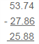Therefore 53.74 – 27.86 = 25.88

(2) 64.98 from 103.87

Let us write the numbers in the column form with the larger one at the top.

Now, subtracting: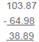Therefore 103.87 – 64.98 = 38.89

(3) 59.63 from 92.4

Converting the given numbers into like decimals:

59.63 and 92.40

Let us write them in the column form with the larger number at the top.

Now, subtracting: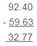Therefore 92.4 – 59.63 = 32.77

(4) 56.8 from 204

Converting the given numbers into like decimals:

56.80 and 204.00

Let us write them in the column form with the larger number at the top.

Now, subtracting: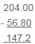Therefore 204.00 – 56.80 = 147.2

(5) 127.38 from 92.4

Converting the given numbers into like decimals:

127.38 and 216.20

Let us write them in the column form with the larger number at the top.

Now, subtracting: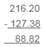Therefore, 216.20 – 127.38 = 88.82

(6) 39.875 from 70.68

Converting the given numbers into like decimals:

39.875 and 70.680

Let us write them in the column form with the larger number at the top.

Now, subtracting: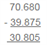Therefore, 70.680 – 39.875 = 30.805

(7) 348.237 from 523.12

Converting the given numbers into like decimals:

348.237 and 523.120

Let us write them in the column form with the larger number at the top.

Now, subtracting: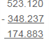Therefore, 523.120 – 348.237 = 174.883

(8) 485.573 from 600

Converting the given numbers into like decimals:

458.573 and 600.000

Let us write them in the column form with the larger number at the top.

Now, subtracting: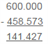Therefore, 600.000 – 458.573 = 141.427

(9) 149.456 from 206.321

Let us write them in the column form with the larger number at the top.

Now, subtracting: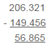Therefore, 206.321 – 149.456 = 56.865

(10) 0.612 from 3.4

Converting the given numbers into like decimals:

3.400 and 0.612

Let us write them in the column form with the larger number at the top.

Now, subtracting: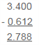Therefore, 3.400 – 0.612 = 2.788

Simplify:

(11) 37.6 + 72.85 – 58.678 – 6.09

Converting the given decimals into like decimals, then adding and, finally, subtracting: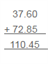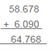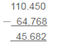(37.60 + 72.85) – (58.678 + 6.090)

= 110.450 – 64.768

= 45.682

(12) 75.3 – 104.645 + 178.96 – 47.9

Converting the given decimals into like decimals, then adding and, finally, subtracting: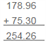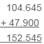(75.30 + 178.96) – (104.645 + 47.900)

245.260 – 152.545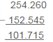101.715

(13) 213.4 – 56.84 – 11.87 – 16.087

Converting the given decimals into like decimals, then adding and, finally, subtracting: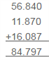(213.400 ) – (56.840 + 11.870 + 16.087)

213.400 – 84.797

128.603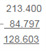(14) 76.3 – 7.666 – 6.77

Converting the given decimals into like decimals, then adding and, finally, subtracting: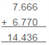(76.300) – (7.666 + 6.770)

= 76.300 – 14.436

= 61.864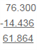(15) What is to be added to 74.5 to get 91?

In order to get the number that must be added to 74.5 to get 91, we must subtract 74.5 from 91.0.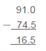Thus, 16.5 is the required number.

(16) What is to be subtracted from 7.3 to get 0.862?

In order to get the number that must be subtracted from 7.300 to get 0.0862, we have to subtract 0.862 from 7.300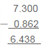Thus, 6.438 is the required number.

(17) By how much should 23.754 be increased to get 50?

In order to get the number by which 23.754 must be increased to get 50, we have to subtract 23.754 from 50.000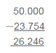(18) By how much should 84.5 be decreased to get 27.84?

In order to get the number by which 84.50 must be decreased to get 27.84, we have to subtract 27.84 from 84.50.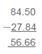(19) If the school bag of Neelam and Garima weigh 6 kg 80 g and 5 kg 265 g respectively, whose bag is heavier and how much?

Weight of Neelam’s bag = 6080 g {converting into grams: 6 kg + 80 g = (6000 + 80) g = 6080 g}

Weight of Garima’s bag = -5265 g {converting into grams: 5 kg + 265 g = (5000 + 265) g = 5265 g}

Difference of the weight of bag = 815 g

Thus, the weight of Neelam’s school bag is more than that of Garima’s school bag by 815 gram, i.e. by 0.815 kg.

(20) Kunal purchased a notebook for Rs 19.75, a pencil for Rs 3.85 and a pen for Rs 8.35 from a book shop. He gave a 50 Rs note to the shopkeeper. What amount did he get back?

Cost of notebook = Rs 19.75

Cost of pencil = Rs 3.85

Cost of pen = + Rs 8.35

Total cost payable = Rs31.95

Total money paid = Rs 50.00

Total money spent= – Rs 31.95

Balance = Rs 18.05

Thus, Kunal got back Rs 18.05 from the shopkeeper.

(21) Sunita purchased 5 kg 75 g of fruit and 3 kg 465 g of vegetables, and put them in a bag. If this bag with these contents weight 9 kg, find the weight of the empty bag.

Weight of the fruits = 5 kg 075 g

Weight of the vegetables = + 3 kg 465 g

Total weight of the content of the bag = 8 kg 540 g

Total weight of the bag with its content = 9 kg 000 g

Total weight of the contents of the bag = – 8 kg 540 g

Weight of the empty bag = 0 kg 460 g

Thus, the weight of the empty bag is 460 grams.

22. The distance between Reeta’s house and her office is 14 km. She covers 10 km 65 m by scooter. 3 km 75 m by bus and the rest on foot. How much distance does she cover by walking?

Converting into meters:

10 km 65 m = (10 + 0.065) m = 10.065 m

3 km 75 m = (3 + 0.075) m = 3.075 m

Distance covered by the scooter = 10.065 km

Distance covered by the bus = +3.075 km

Total distance covered by the bus and the scooter = 13.140 km

Total distance between the house and the office = 14.000 km

Total distance covered by the bus and the scooter = -13.140 km

Distance covered on foot = 0.860 km

Therefore, distance covered by walking = 0.860 km = 860 meters.

Exercise 7E

OBJECTIVE QUESTIONS

Mark correct against the correct answer in each of the following:

(1) $\frac {7} {10} = ?$

(a) 7.1 (b) 1.7 (c) 0.7 (d) 0.07

(c) 0.7

$\frac {7} {10} = 7\; tenths = 0.7$

(2) $\frac {5} {100} = ?$

(a) 5.1 (b) 5.01 (c) 0.5 (d) 0.05

(d) 0.05

$\frac {5} {100} = 5 \; hundredths = 0.05$

(3) $\frac {9} {1000} = ?$

(a) 0.0009 (b) 0.009 (c) 9.001 (d) none of these

(b) 0.009

$\frac {9} {1000} = 9 \; thousandth = 0.009$

(4) $\frac {16} {1000} = ?$

(a) 0.016 (b) 0.16 (c) 0.0016 (d) 1.006

(a) 0.016

$\frac {16} {1000} = 16 \; thousandths = 0.016$

(5) $\frac {134} {1000} = ?$

(a) 13.4 (b) 1.34 (c) 0.134 (d) 0.0134

(c) 0.134

$\frac {134} {1000} = 134 \; thousandths = 0.134$

(6) $2 \frac {17} {100} = ?$

(a) 2.17 (b) 2.017 (c) 0.217 (d) 21.7

(a) 2.17

$2\frac{17}{100} = 2 + \frac{17}{100} = 2 + 0.17 = 2.17$

(7) $4 \frac {3} {100} = ?$

(a) 4.3 (b) 4.03 (c) 4.003 (d) 43.10

(b) 4.03

$4 \frac {3} {100} = 4 + \frac {3} {100} = 4 + 0.03 = 4.03$

(8) 6.25 =?

(a) $6 \frac {1} {2}$

(b) $6 \frac {1} {4}$

(c) $62 \frac {1} {2}$

(d) none of these

(b) $6.25 = 6 + 0.25 = 6 + = 6 + \frac {1} {4} = 6 \frac {1} {4}$

(9) $\frac {6} {25} = ?$

(a) 2.4 (b) 0.24 (c) 0.024 (d) none of these

(b) 0.24

$\frac {6} {25} = 0.24$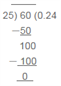(10) $4 \frac {7} {8} = ?$

(a) 4.78 (b) 4.87 (c) 4.875 (d) none of these

(c) 4.875

$4 \frac {7} {8} = 4 + \frac {7} {8} = 4 + 0.875 = 4.875$

(11) 24.8 = ?

(a) $24 \frac {4} {5}$

(b) $24 \frac {2} {5}$

(c) $24 \frac {1} {5}$

(d) none of these

(c) $24 \frac {4} {5}$

$24.8 = 24 + 0.8 = 24 + \frac {8} {10} = 24 + \frac {4} {5} = 24 \frac {4} {5}$

(12) $2 \frac {1} {25} = ?$

(a) 2.4 (b) 2.04 (c) 2.004 (d) none of these

(b) 2.04

$2 \frac {1} {25} = 2 + \frac {1} {25} = 2 + 0.04 = 2.04$

(13) $2 + \frac {3} {10} + \frac {4} {100} = ?$

(a) 2.304 (b) 2.403 (c) 2.34 (d) none of these

(c) 2.34

$2 + \frac {3} {10} + \frac {4} {100} = 2 + 0.3 + 0.04 = 2.34$

(14) $2 + \frac {6} {100} = ?$

(a) 2.006 (b) 2.06 (c) 2.6 (d) none of these

(b) 2.06

$2 + \frac {6} {100} = 2 + 0.06 = 2.06$

(15) $\frac {4} {100} + \frac {7} {10000} = ?$

(a) 0.47 (b) 0.407 (c) 0.0407 (d) none of these

(c) 0.0407

$\frac {4} {100} + \frac {7} {10000} = 0.04 + 0.0007 = 0.0407$

(16) The correct expanded form of 2.06 is

(a) $\left ( 2 \times 10 \right ) + \left ( 6 \times \frac {1} {10} \right )$

(b) $\left ( 2 \times 1 \right ) + \left ( 6 \times \frac {1} {10} \right )$

(c) $\left ( 2 \times 1 \right ) + \left ( 6 \times \frac {1} {100} \right )$

(d) none of these

(c) $\left ( 2 \times 1 \right ) + \left ( 6 \times \frac {1} {100} \right )$

2.06 = 2 + $\frac {6} {100} \left ( 2 \times 1 \right ) + \left ( 6 \times \frac {1} {100} \right )$

(17) Among 2.6, 2.006, 2.66 and 2.08, the largest number is

(a) 2.006 (b) 2.08 (c) 2.6 (d) 2.66

(d) 2.66

Converting the given decimals into like decimals:

2.600, 2.006, 2.660 and 2.080

Among the given decimals, 2.660 is the largest.

(18) Which of the following is the correct order?

(a) 2.2 < 2.02 < 2.002 < 2.222

(b) 2.002 < 2.02 < 2.2 < 2.222

(c) 2.02 < 2.22 < 2.002 < 2.222

(d) none of these

(b) 2.002 < 2.02 < 2.2 < 2.222

Converting the given decimals into like decimals:

2.002, 2.020, 2.200, 2.222

Therefore, 2.002 < 2.02 < 2.2 < 2.222

(19) Which is larger: 2.1 or 2.055?

(a) 2.1 (b) 2.055 (c) cannot be compared

(a) 2.1

If we convert the given decimals into like decimals, we get 2.100 and 2.055. At tenths place, 1 is greater than 0. Thus, 2.100 is greater than 2.055.

(20) 1 cm =?

(a) 0.1 m (b) 0.01 m (c) 0.001 m (d) none of these

(b) 0.01 m

1 m = 100 cm

Therefore, $1\; cm = \frac {1} {100} m = 0.01 m$

(21) 2 m 5 cm =?

(a) 2.5 m (b) 2.05 m (c) 2.005 m (d) 0.25

(b) 2.05 m

2 m 5 cm = $\left ( 2 + \frac {5} {100} \right ) m = \left ( 2 + 0.05 \right ) m = 2.05\; m$

(22) 2 kg 8 g =?

(a) 2.8 kg (b) 2.08 kg (c) 2.008 kg (d) none of these

(c) 2.008 kg

1 kg = 1000 kg

Therefore, 2 kg 8 g = $2\; kg + \frac {8} {1000} \; kg = \left ( 2 + 0.008 \right ) \; kg = 2.008 \; kg$

(23) 2 kg 56 g =?

(a) 2.56 kg (b) 2.056 kg (c) 2.560 kg (d) none of these

(b) 2.056 kg

2 kg + 56 g = $\left ( 2 + \frac {56} {1000} \right ) kg\; = \left ( 2 + 0.056 \right ) \; kg = 2.056 \; kg$

(24) 2 km 35 m =?

(a) 2.35 km (b) 2.350 km (c) 2.035 km (d) none of these

(c) 2.035 km

1 km = 1000 m

Therefore, 2 km 35 m = $\left ( 2 + \frac {35} {1000} \right ) km\; = \left ( 2 + 0.035 \right ) \; km = 2.035 \; km$

(25) 0.4 + 0.004 + 4.4 =?

(a) 4.444 (b) 5.2 (c) 4.804 (d) 5.404

(c) 4.804

0.4 + 0.004 + 4.4

Converting into like decimals and then adding: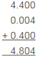(26) 3.5 + 4.05 – 6.005 =?

(a) 1.545 (b) 1.095 (c) 1.6 (d) none of these

(a) 1.545

Converting into like decimals:

3.500 + 4.050 – 6.005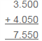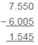(27) 6.3 – 2.8 =?

(a) 0.35 (b) 3.5 (c) 3.035 (d) none of these

(b) 3.5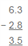(28) 5.01 – 3.6 =?

(a)4.65(b) 1.95 (c) 1.41 (d) none of these

(c) 1.41

Converting into like decimals and then subtracting: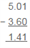(29) 2 – 0.7 =?

(a) 1.3 (b) 1.5 (c) 2.03 (d) none of these

(a) 1.3

Converting into like decimals and then subtracting: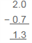(30) 1.1 – 0.3 =?

(a) 0.8 (b) 0.08 (c) 8 (d) none of these

(a) 0.8

Converting into like decimals and then subtracting: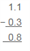#### Practise This Question

The given table shows data for a specific school in India. The State-wise number of students are given.

Calculate the average number of students taking all the regions into consideration.